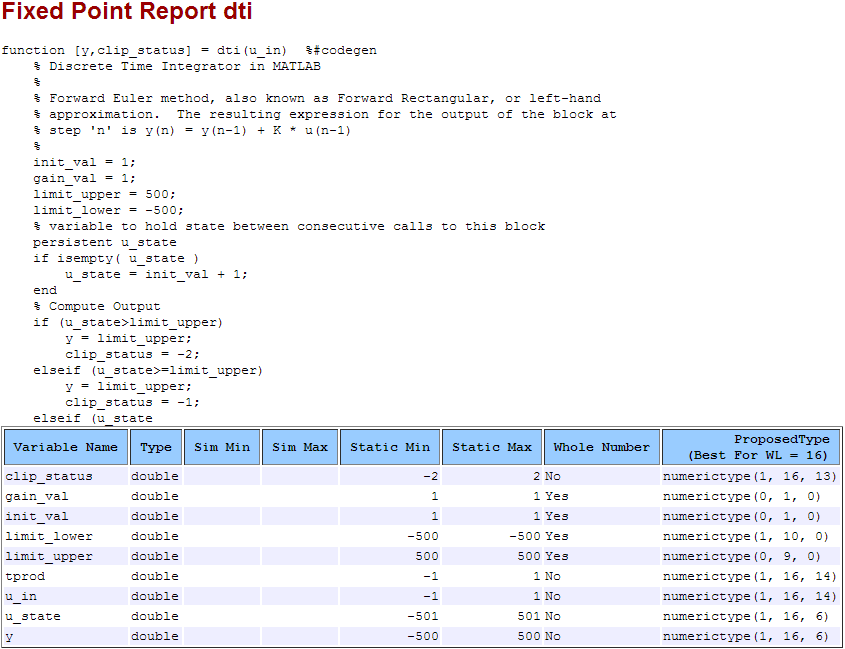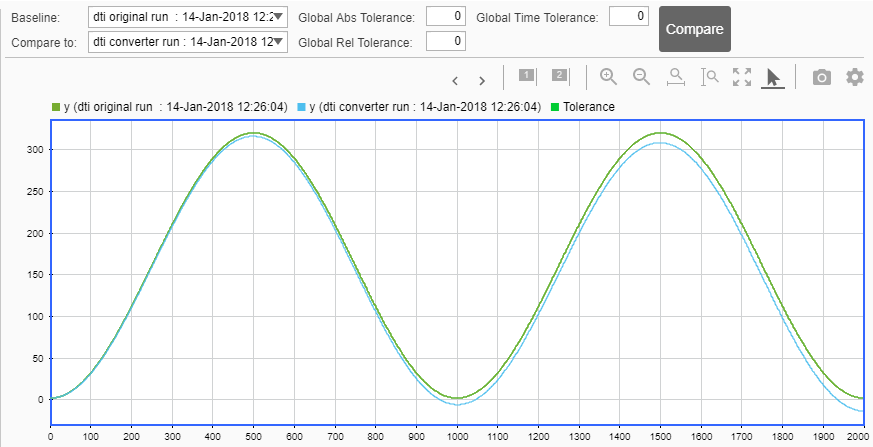Main Content

## Propose Data Types Based on Derived Ranges

This example shows how to propose fixed-point data types based on static ranges using the `fiaccel` function. The advantage of proposing data types based on derived ranges is that you do not have to provide test files that exercise your algorithm over its full operating range. Running such test files often takes a very long time so you can save time by deriving ranges instead.

Note

Derived range analysis is not supported for non-scalar variables.

### Prerequisites

To complete this example, you must install the following products:

• MATLAB®

• Fixed-Point Designer™

• C compiler

You can use `mex -setup` to change the default compiler. See Change Default Compiler.

### Create a New Folder and Copy Relevant Files

1. Create a local working folder, for example, `c:\dti`.

2. Change to the `docroot\toolbox\fixpoint\examples` folder. At the MATLAB command line, enter:

`cd(fullfile(docroot, 'toolbox', 'fixpoint', 'examples')) `

3. Copy the `dti.m` and `dti_test.m` files to your local working folder.

It is best practice to create a separate test script to do all the pre- and post-processing such as loading inputs, setting up input values, calling the function under test, and outputting test results.

TypeNameDescription
Function code`dti.m`Entry-point MATLAB function
Test file`dti_test.m`MATLAB script that tests `dti.m`

### Set Up the Fixed-Point Configuration Object

Create a fixed-point configuration object and configure the test file name.

```fixptcfg = coder.config('fixpt'); fixptcfg.TestBenchName = 'dti_test'; ```

### Specify Design Ranges

Specify design range information for the `dti` function input parameter `u_in`.

```fixptcfg.addDesignRangeSpecification('dti', 'u_in', -1.0, 1.0) ```

### Enable Plotting Using the Simulation Data Inspector

Select to run the test file to verify the generated fixed-point MATLAB code. Log inputs and outputs for comparison plotting and select to use the Simulation Data Inspector to plot the results.

```fixptcfg.TestNumerics = true; fixptcfg.LogIOForComparisonPlotting = true; fixptcfg.PlotWithSimulationDataInspector = true;```

### Derive Ranges and Generate Fixed-Point Code

Use the `fiaccel` function to convert the floating-point MATLAB function, `dti`, to fixed-point MATLAB code. Set the default word length for the fixed-point data types to 16.

```fixptcfg.ComputeDerivedRanges = true; fixptcfg.ComputeSimulationRanges = false; fixptcfg.DefaultWordLength = 16; % Derive ranges and generate fixed-point code fiaccel -float2fixed fixptcfg dti```

`fiaccel` analyzes the floating-point code. Because you did not specify the input types for the `dti` function, the conversion process infers types by simulating the test file. The conversion process then derives ranges for variables in the algorithm. It uses these derived ranges to propose fixed-point types for these variables. When the conversion is complete, it generates a type proposal report.

### View Derived Range Information

Click the link to the type proposal report for the `dti` function, `dti_report.html`.

The report opens in a web browser.### View Generated Fixed-Point MATLAB Code

`fiaccel` generates a fixed-point version of the `dti` function, `dti_fxpt.m`, and a wrapper function that calls `dti_fxpt`. These files are generated in the `codegen\dti\fixpt` folder in your local working folder.

```function [y, clip_status] = dti_fixpt(u_in) %#codegen % Discrete Time Integrator in MATLAB % % Forward Euler method, also known as Forward Rectangular, or left-hand % approximation. The resulting expression for the output of the block at % step 'n' is y(n) = y(n-1) + K * u(n-1) % fm = get_fimath(); init_val = fi(1, 0, 1, 0, fm); gain_val = fi(1, 0, 1, 0, fm); limit_upper = fi(500, 0, 9, 0, fm); limit_lower = fi(-500, 1, 10, 0, fm); % variable to hold state between consecutive calls to this block persistent u_state; if isempty(u_state) u_state = fi(init_val+fi(1, 0, 1, 0, fm), 1, 16, 6, fm); end % Compute Output if (u_state > limit_upper) y = fi(limit_upper, 1, 16, 6, fm); clip_status = fi(-2, 1, 16, 13, fm); elseif (u_state >= limit_upper) y = fi(limit_upper, 1, 16, 6, fm); clip_status = fi(-1, 1, 16, 13, fm); elseif (u_state < limit_lower) y = fi(limit_lower, 1, 16, 6, fm); clip_status = fi(2, 1, 16, 13, fm); elseif (u_state <= limit_lower) y = fi(limit_lower, 1, 16, 6, fm); clip_status = fi(1, 1, 16, 13, fm); else y = fi(u_state, 1, 16, 6, fm); clip_status = fi(0, 1, 16, 13, fm); end % Update State tprod = fi(gain_val * u_in, 1, 16, 14, fm); u_state(:) = y + tprod; end function fm = get_fimath() fm = fimath('RoundingMethod', 'Floor', 'OverflowAction', 'Wrap', 'ProductMode', 'FullPrecision', 'MaxProductWordLength', 128, 'SumMode', 'FullPrecision', 'MaxSumWordLength', 128); end ```

### Compare Floating-Point and Fixed-Point Runs

Because you selected to log inputs and outputs for comparison plots and to use the Simulation Data Inspector for these plots, the Simulation Data Inspector opens.

You can use the Simulation Data Inspector to view floating-point and fixed-point run information and compare results. For example, to compare the floating-point and fixed-point values for the output `y`, on the Compare tab, select `y`, and then click Compare Runs.

The Simulation Data Inspector displays a plot of the baseline floating-point run against the fixed-point run and the difference between them.## SupportGet trial now